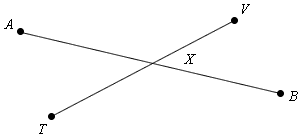Ask Remember Me?kjhgfdsa2 Posts: 1, Reputation: 1 New Member #1 Jan 5, 2009, 01:00 PM
Geometry two column proofs
Write a two-column proof.

If m ∠ 2 ≅ m ∠ 3 then m &InvisibleTimes; ∠ 3 ≅ m ∠ 2
Given: A B ≅ T V and X B ≅ X T

Prove: A X ≅ V XEuRa Posts: 315, Reputation: 64 Full Member #2 Jan 7, 2009, 07:09 PM

Which is angle 2 and 3? No matter, you can still solve it.

I don't want to flat out give you the answer, but first you need to mention that if you have two lines of equal length, and if you subtract 2 similar lines (respectively) of equal length, you will be left with 2 lines of equal length, thus proving what you need to prove. Should only take you 2-3 additional steps, depending on the method of which you choose.

 Question Tools Search this QuestionShow Printable VersionEmail this Page Search this Question: Advanced Search

## Check out some similar questions!

Geometric Proofs [ 1 Answers ]

I need help solving my geometric proofs I need step by step instructions that I can apply to any proof.

Pre cal proofs [ 2 Answers ]

can someone please help me slove these 4 questionss? 1) Sin/ Csc + Cos/ Sec =1 2) sin^2/Sec^2-1 = Cos^2

Precal proofs [ 1 Answers ]

can someone please help me slove these 4 questionss? 1) Sin/ Csc + Cos/ Sec =1 2) sin^2/Sec^2-1 = Cos^2

I created an alphabetized column with names. In another column I put an x next to those names that were heads of households leaving the rest blank. I would like to create a new column that would recognize the x then insert a number in the cell next to the x in descending order (i.e. 1-50).